# Planimetrics - math word problems

Study plane measurements, including angles, distances, and areas. In other words - measurement and calculation of shapes in the plane. Perimeter and area of plane shapes.

#### Number of problems found: 1947

• The chordCalculate a chord length which the distance from the center of the circle (S, 6 cm) equals 3 cm.
• RT and circlesSolve right triangle if the radius of inscribed circle is r=9 and radius of circumscribed circle is R=23.
• Chord - TSThe radius of circle k measures 68 cm. Arc GH = 47 cm. What is TS?
• Body diagonalCalculate the volume and surface of the cube if the body diagonal measures 10 dm.
• Inscribed circle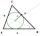The circle inscribed in a triangle has a radius 3 cm. Express the area of the triangle using a, b, c.
• Carpet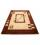What is the price of square carpet side length 3.25 m if one meter square cost 230 CZK?
• Trapezoid ABCD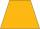Calculate the perimeter of trapezoid ABCD if we know the side c=15, b=19 which is also a height and side d=20.
• Square sides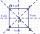If we enlarge the side of the square by a third, its circumference will be 20 cm larger than the original. What is the side of the square?
• The aspect ratioThe aspect ratio of the rectangular triangle is 13: 12: 5. Calculate the internal angles of the triangle.
• Vertex pointsGiven the following points of a triangle: P(-12,6), Q(4,0), R(-8,-6). Graph the triangle. Find the triangle area.
• Rhombus diagonalArea of rhombus is 224. One diagonal measures 33, find length of other diagonal.
• Vectors abs sum diff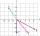The vectors a = (4,2), b = (- 2,1) are given. Calculate: a) |a+b|, b) |a|+|b|, c) |a-b|, d) |a|-|b|.
• Diamond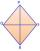Calculate the length of the two diagonals of the diamond if: a = 13 cm v = 12 cm
• Forces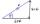Determine the resultant of two perpendicular forces F1 = 560 N and second force of 25% smaller.
• DecagonCalculate the area and circumference of the regular decagon when its radius of a circle circumscribing is R = 1m
• It is 2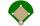It is 90 feet from home plate to first base on a baseball diamond. What is the area of the baseball diamond in square yards?
• Argicultural fieldField has a rectangular shape with a width 180 m and circumference 940 m. How many hectares acreage has field?
• LandLand has a rectangular shape, its surface area is 1.45 hectares. Its width is 250 m. Determine the length of the land.
• BaseCompute base of an isosceles triangle, with the arm a=20 cm and a height above the base h=10 cm.
• Square sides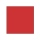If the square side increases by 28%, the square circumference increases by 11.2 meters. Find the length of the original square side.

Do you have an interesting mathematical word problem that you can't solve it? Submit a math problem, and we can try to solve it.

We will send a solution to your e-mail address. Solved examples are also published here. Please enter the e-mail correctly and check whether you don't have a full mailbox.

Please do not submit problems from current active competitions such as Mathematical Olympiad, correspondence seminars etc...